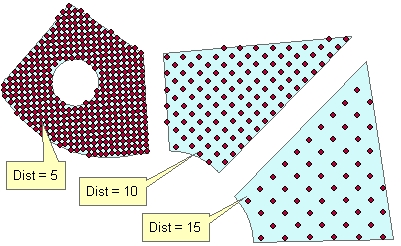Go to ET GeoWizards 12.x User Guide

Point Grids In Polygons

Go to ToolBox Implementation

Generates uniform (regularly spaced) points located in the polygons of the input polygon dataset. The distance between the points for each polygon can be the same or different - based on the values in a numeric field of the input polygon feature class. The user can specify the rotation angle for the resulting point grid.

Inputs:

• A polygon feature class
• The distance between the points for each polygon can be input in one of the following ways
• A numeric field which values will be used to get the distance between points for each polygon.
• A constant number defining the distance between points for all polygons.
• Rotation angle
• Constant for all polygons - (in degrees starting from East anti-clockwise)
• From field - different rotation angle for each field (in degrees starting from East anti-clockwise)
• Along the longest axis of each polygon
• Along the longest side of each polygon

Outputs:

• New Point feature class.
• The following fields are added to the attribute table of the resulting feature class.
• [ET_ID] - the ID of the original polygon

Illustration:

 Distance between points = 10, Rotational angle = 0Original polygons labeled with the values in field to be used as a source for getting the distance between the points. Rotational angle = "Along the longest Side"Command line syntax

ET_GPPointGridsInPolygons<input_dataset>  <out_feature_class> {distance} {distance_field} {angle_from} {rotation_angle} {angle_field}

Parameters

Expression Explanation
<input_dataset> A Polygon feature class or feature layer
<out_feature_class> A String - the full name of the output feature class.
{distance} A Double representing the distance between the points for all polygons
{distance_field}  A String representing the name of a numeric field which values will be used to get the distance between the points for each polygon
{angle_from} A String defining how the rotation angle will be specified
• "Constant" - Constant for all polygons - (in degrees starting from East anti-clockwise)
• "From field" - different rotation angle for each field (in degrees starting from East anti-clockwise)
• "Longest Axis" - Along the longest axis of each polygon
• "Longest Side" - Along the longest side of each polygon
{rotation_angle} A Double - specifying the rotation angle for all polygons.
{angle_field} A String - the name of the field to be used as source for rotation angle.

Scripting syntax

ET_GPPointGridsInPolygons(input_dataset,  out_feature_class, distance, distance_field,  angle_from, rotation_angle, angle_field)

See the explanations above:
<> - required parameter
{} - optional parameter